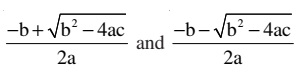Quadratic polynomial is a polynomial of degree 2. Quadratic equation is an equation having degree 2. General form of a quadratic equation is ax2 + bx + c = 0, a ≠ 0 where a, b, c are real numbers and x is a variable.

Roots of a quadratic equation: Values of variable which satisfy a quadratic equation. α is a root of the quadratic equation if aα2 + bα + c = 0. A quadratic equation has two roots. Zeros of a quadratic polynomial and the roots of the corresponding quadratic equation are the same.

Methods for solution of quadratic equation:

1. Factor method

Factor method: Factorise ax2 + bx + c , a ≠ 0 into a product of two linear factors. Equate each factor to zero and get the values of the variable. These values are the required roots of the given quadratic equation.

Quadratic formula: The roots of the equation are:Discriminant: The expression b2 - 4ac is called discriminant of the equation ax2 + bx +c = 0 and denoted by D.

Nature of Roots: A quadratic equation ax2 + bx + c = 0 (a ≠ 0) has

1. two distinct real roots if D > 0
2. two equal (or coincident) and real roots if D = 0
3. no real root if D = < 0

Word Problems or daily life problems: To solve a word problem using quadratic equations convert the given problem in the form of a quadratic equation and then solve the equation by using factor method or quadratic formula.